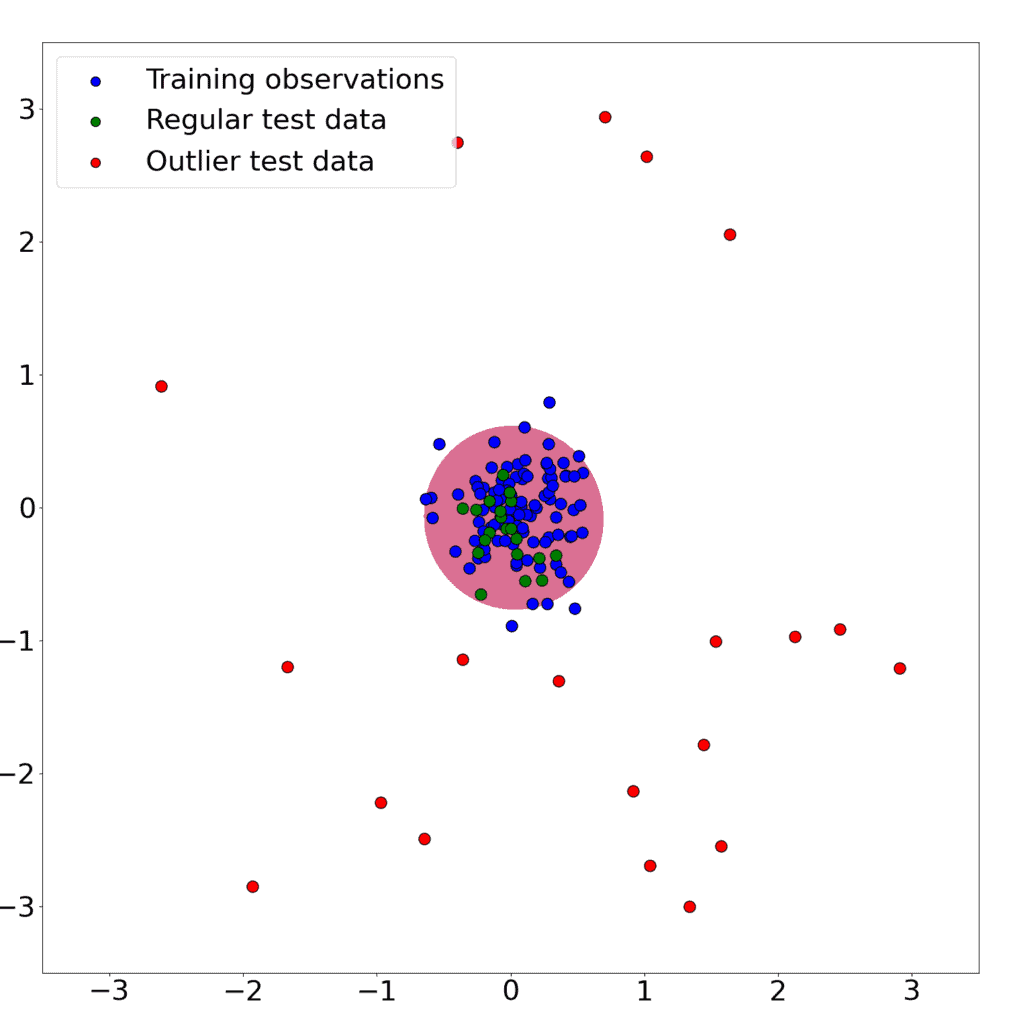## 1. Introduction

One Class Support Vector Machines (SVMs) are a type of outlier detection method.

In this tutorial, we’ll explore how SVMs perform outlier detection and illustrate its utility with a simple example.

## 2. Support Vector Machines

Usually, SVMs aim to generalize from some input data. With this, they can decide whether any additional data are outliers or not.

Basic SVMs do this by separating data into several classes. From there, they can decide which class any subsequent data belongs to.

One Class SVMs are similar, but there is only one class. Therefore, a boundary is decided on using the available data. Any new data that lies outside that boundary is classed as an outlier.

To see how this is done, let’s dive into the formulation.

## 3. One Class SVM

There are two formulations of One Class SVMs. The first is by Schölkopf, which involves using a hyperplane (a plane in n-dimensions) for the decision boundary. The second is by Tax and Duin, which uses a hypersphere for the decision boundary. Let’s explore the latter.

The hypersphere is characterized by a centerwith a radius. Also, let’s say we havedata points that are given by, where.

Therefore, the Euclidean distance from the hypersphere center and a given data point is. We want to minimize a cost functionwith the constraint that every point lies within or on the hypersphere. Therefore, our constraint is.

As it stands, outliers will greatly affect the tuning. Let’s address this by modifying the cost function toand the constraint to.are the positive weights associated with each data point. The higher it is, the less that particular data point affects the tuning of. Also,provides a trade-off between volume and classification errors.

Combining this with the method of Lagrange Multipliers, we are left with an optimization problem to solve:

(1)whereandare non-negative Lagrange multipliers.should be maximized with respect toand, but minimized with respect toand.

Now let’s apply this to a simple problem!

## 4. Simple Example

Suppose we train a One Class SVM on 100 data points from a two-dimensional Gaussian distribution.

After that, we add both 20 additional data points and 20 data points drawn from a Uniform distribution. Our goal is to correctly classify the Gaussian data points while ignoring the outlier data from the Uniform distribution.

This is the result:## 5. Conclusion

In this brief article, we showed how they differ from basic SVMs. Then, we outlined the relevant equations and applied them to a simple example.